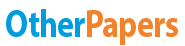Essay by   •  April 26, 2018  •  Exam  •  1,284 Words (6 Pages)  •  339 Views

## Essay Preview: Statistic Exam Question Answers

Report this essay
Page 1 of 6
1. (5%) Given: The probabilities of three events, A, B, and C, occurring are

P(A) = 0.35, P(B) = 0.45, and P(C) = 0.2. Assuming that A, B, or C has

occurred, the probabilities of another event, X, occurring are P(X│A)=0.8,

P(X│B)=0.65, and P(X│C)=0.3. Find P(A│X)= _____________.

2. (5%) A Ph.D. graduate has applied for a job with two universities: A and B.

The graduate feels that she has a 60% chance of receiving an offer from

university A and a 50% chance of receiving an offer from university B. If she

receives an offer from university B, she believes that she has an 80% chance

of receiving an offer from university A. What is the probability that at

least one university will make her an offer?

Probability = _________________

3. (5%) The monthly sales at a bookstore have a mean of 50,000 and a standard

deviation of 6,000. Profits are calculated by multiplying sales by 40% and

subtracting fixed costs of 12,000. Find the standard deviation of monthly profits.

Standard deviation of monthly profits = ____________

4. (5%) Suppose a disease is present in 3% of population. A diagnostic test for

such disease shows 10% false positive and 5% false negative. That is, for a

patient having the disease,, the test shows positive (+) with probability

0.95 and negative (-) with probability 0.05. For a patient not having

disease, the test shows positive (+) with probability 0.10 and negative (-)

with probability 0.90. If a patient’s test show (+), what’s the probability

of his having the disease?

Probability = ______________

5. (6%) Throw a dice n times fairly and observe the number of dice each time.

Assume a random variable X is the frequency that the number of dice is one.

1. (3%) Write down the probability distribution of random variable

b. (3%) Assume n = 5, P(X<=1 or X>=5)=______________

6. (5%) A communication system consists of n components, each of which will,

independently, function with probability p. The total system will be able to

operate effectively if at least one-half of its components function. For what

values of p is a 5-component system more likely to operate effectively than a

3-component system?

Values of p should be ___________

7. (6%) Suppose that earthquake occur in the eastern part of Taiwan in

accordance with the assumptions for the Poisson probability distribution at a

rate of 2 per day.

a. (3%) Find the probability that at least 3 earthquake occur during the next

2 days = _______________

b. (3%) Find the probability distribution of the time, starting from now,

until the next earthquake. _____________________

8. (5%) Suppose that the proportion of colorblind people in a certain

population is 0.005. What is the probability that there will not be more than

one colorblind person in a randomly chosen group of 600 people? You can use

Poisson distribution to approximate the binomial distribution.

Probability = __________________

9. (5%) Suppose the daily amount of waste generated per person is normally

distributed, with mean 3.58 pounds and standard deviation 1.04 pounds. Of the

daily amounts of waste generated per person, 67.72% would be greater than

what amount? ____________________

10. (5%) Suppose that customers arrive at a drive through window at an

average rate of three customers per minute and that their arrival follow the

Poisson model. Use the appropriate distribution to find the probability that

the next customer will arrive within 1.5 minutes.

Probability = ________________

11. (7%) Suppose that patrons of a restaurant were asked whether they preferred

beer or whether they preferred wine. 60% said that they preferred beer. 70% of

the patrons were male. 80% of the males preferred beer.

1. (3%) What is the probability a randomly selected patron prefers wine?

Probability = ____________

b. (3%) Suppose a randomly selected patron is a female. What is the probability

that the patron prefers wine?

Probability = ____________

c. (1%) Are gender of patrons and drinking preference independent?

Choose yes or no = _____________  Explain below.

12. (5%) Suppose that X is a random variable for which E(X)=μ and Var(X)=σ^2.

Find E[X(X-1)]=__________________

13. (5%) Suppose that X and Y are random variables such that Var(X)=9, Var(Y)=4,

and ρ(X,Y)=-1/6. Find Var(X-3Y+4)=______________

14. (5%) The average score of all student in an economics class has a mean of

70 and a standard deviation of 3. Suppose a sample of 36 students took the

class this semester. Find the probability that the average score of the 36

...

...